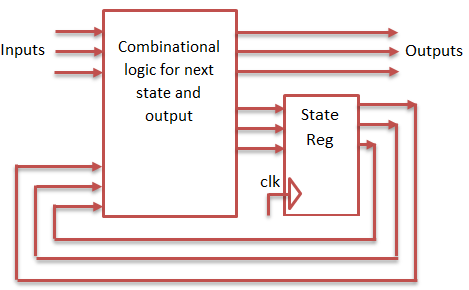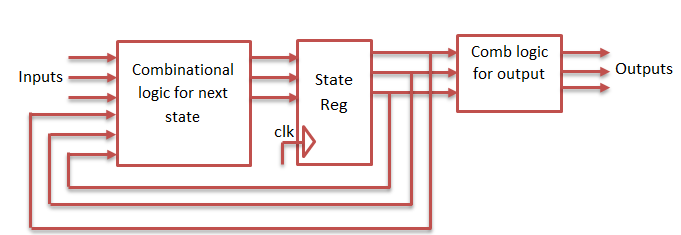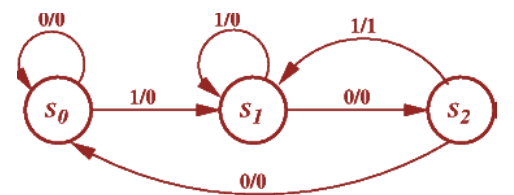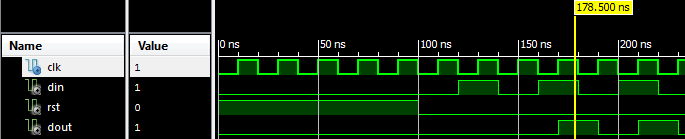# Sequence Detector using Mealy and Moore State Machine VHDL Codes

### Mealy State Machine

The Output of the state machine depends on both present state and current input. When the input changes,the output of the state machine updated without waiting for change in clock input.### Moore State Machine

The Output of the State machine depends only on present state. The output of state machine are only updated at the clock edge.Let’s construct the sequence detector for the sequence 101 using both mealy state machine and moore state machine.

Moore state require to four states st0,st1,st2,st3 to detect the 101 sequence.Mealy state machine require only three states st0,st1,st2 to detect the 101 sequence.### VHDL code for Sequence detector (101) using moore state machine

```library IEEE;
use IEEE.STD_LOGIC_1164.ALL;

entity moore is
Port ( clk : in STD_LOGIC;
din : in STD_LOGIC;
rst : in STD_LOGIC;
dout : out STD_LOGIC);
end moore;

architecture Behavioral of moore is
type state is (st0, st1, st2, st3);
signal present_state, next_state : state;
begin

synchronous_process: process (clk)
begin
if rising_edge(clk) then
if (rst = '1') then
present_state <= st0;
else
present_state <= next_state;
end if;
end if;
end process;

output_decoder : process(present_state, din)
begin
next_state <= st0; case (present_state) is when st0 =>
if (din = '1') then
next_state <= st1;
else
next_state <= st0; end if; when st1 =>
if (din = '1') then
next_state <= st1;
else
next_state <= st2; end if; when st2 =>
if (din = '1') then
next_state <= st3;
else
next_state <= st0; end if; when st3 =>
if (din = '1') then
next_state <= st1;
else
next_state <= st2; end if; when others =>
next_state <= st0; end case; end process; next_state_decoder : process(present_state) begin case (present_state) is when st0 =>
dout <= '0'; when st1 =>
dout <= '0'; when st2 =>
dout <= '0'; when st3 =>
dout <= '1'; when others =>
dout <= '0';
end case;
end process;

end Behavioral;
```

### VHDL code for Sequence detector (101) using mealy state machine

```library IEEE;
use IEEE.STD_LOGIC_1164.ALL;

entity mealy is
Port ( clk : in STD_LOGIC;
din : in STD_LOGIC;
rst : in STD_LOGIC;
dout : out STD_LOGIC);
end mealy;

architecture Behavioral of mealy is
type state is (st0, st1, st2, st3);
signal present_state, next_state : state;
begin

syncronous_process : process (clk)
begin
if rising_edge(clk) then
if (rst = '1') then
present_state <= st0;
else
present_state <= next_state;
end if;
end if;
end process;

next_state_and_output_decoder : process(present_state, din)
begin
dout <= '0'; case (present_state) is when st0 =>
if (din = '1') then
next_state <= st1;
dout <= '0';
else
next_state <= st0;
dout <= '0'; end if; when St1 =>
if (din = '1') then
next_state <= st1;
dout <= '0';
else
next_state <= st2;
dout <= '0'; end if; when St2 =>
if (din = '1') then
next_state <= st1;
dout <= '1';
else
next_state <= st0;
dout <= '0'; end if; when others =>
next_state <= st0;
dout <= '0';
end case;
end process;

end Behavioral;
```

### TestBench VHDL code for sequence detector using Moore State Machine

```
LIBRARY ieee;
USE ieee.std_logic_1164.ALL;

ENTITY tb_moore IS
END tb_moore;

ARCHITECTURE behavior OF tb_moore IS

-- Component Declaration for the Unit Under Test (UUT)

COMPONENT moore
PORT(
clk : IN std_logic;
din : IN std_logic;
rst : IN std_logic;
dout : OUT std_logic
);
END COMPONENT;

--Inputs
signal clk : std_logic := '0';
signal din : std_logic := '0';
signal rst : std_logic := '0';

--Outputs
signal dout : std_logic;

-- Clock period definitions
constant clk_period : time := 20 ns;

BEGIN

-- Instantiate the Unit Under Test (UUT)
uut: moore PORT MAP (
clk => clk,
din => din,
rst => rst,
dout => dout
);

-- Clock process definitions
clk_process :process
begin
clk <= '0';
wait for clk_period/2;
clk <= '1';
wait for clk_period/2;
end process;

-- Stimulus process
stim_proc: process
begin

rst <= '1';

wait for 100 ns;

rst <= '0';

din <= '0';

wait for 20 ns;

din <= '1';

wait for 20 ns;

din <= '0';

wait for 20 ns;

din <= '1';

wait for 20 ns;

din <= '0';

wait for 20 ns;

din <= '1';

wait for 20 ns;

din <= '0';

wait for 20 ns;

din <= '1';
end process;

END;

```

Note: Same testbench code can be used for Mealy VHDL code by simply changing the component name to mealy.

### TestBench output waveform for Mealy and Moore State MachineFrom the above shown waveform, sequence 101 is detected twice from the testbench VHDL code.

### 3 thoughts on “Sequence Detector using Mealy and Moore State Machine VHDL Codes”

1.In the moore type FSM code, shouldn’t it be the next_state decoder first and then the output_decoder (the names’ order)?

2.Thanks, very helping. this working

3.